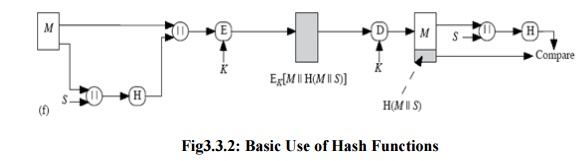# Hash Functions

A variation on the message authentication code is the one way hash function. As with MAC, a hash function accepts a variable size message M as input and produces a fixed-size output, referred to as hash code H(M).

HASH FUNCTIONS

A variation on the message authentication code is the one way hash function. As with MAC, a hash function accepts a variable size message M as input and produces a fixed-size output, referred to as hash code H(M). Unlike a MAC, a hash code does not use a key but is a function only of the input message. The hash code is also referred to as a message digest or hash value.

There are varieties of ways in which a hash code can be used to provide message authentication, as follows:

a) The message plus the hash code is encrypted using symmetric encryption. This is identical to that of internal error control strategy. Because encryption is applied to the entire message plus the hash code, confidentiality is also provided.b)      Only the hash code is encrypted, using symmetric encryption. This reduces the processing burden for those applications that do not require confidentiality.c)       Only the hash code is encrypted, using the public key encryption and using the sender‟s private key. It provides authentication plus the digital signature.

If confidentiality as well as digital signature is desired, then the message plus the public key encrypted hash code can be encrypted using a symmetric secret key.This technique uses a hash function, but no encryption for message authentication. This technique assumes that the two communicating parties share a common secret value „S‟. The source computes the hash value over the concatenation of M and S and appends the resulting hash value to M.

Confidentiality can be added to the previous approach by encrypting the entire message plus the hash code.A hash value h is generated by a function H of the form

h = H(M)

where M is a variable-length message and H(M) is the fixed-length hash value. The hash value is appended to the message at the source at a time when the message is assumed orknown to be correct. The receiver authenticates that message by recomputing the hashvalue.

Requirements for a Hash Function

a.     H can be applied to a block of data of any size.

b.     H produces a fixed-length output.

c.      H(x) is relatively easy to compute for any given x, making both hardware and software implementations practical.

d.     For any given value h, it is computationally infeasible to find x such that H(x) = h. This is sometimes referred to in the literature as the one-way property.

e.      For any given block x, it is computationally infeasible to find y, x such that H(y) = H(x). This is sometimes referred to as weak collision resistance.

f.       It is computationally infeasible to find any pair (x, y) such that H(x) = H(y). This is sometimes referred to as strong collision resistance.

The first three properties are requirements for the practical application of a hash function to message authentication.

The fourth property, the one-way property, states that it is easy to generate a code given a message but virtually impossible to generate a message given a code. The fifth property guarantees that an alternative message hashing to the same value as a given message cannot be found. This prevents forgery when an encrypted hash code is used ( Figures b and c).

The sixth property refers to how resistant the hash function is to a type of attack known as the birthday attack, which we examine shortly.

Study Material, Lecturing Notes, Assignment, Reference, Wiki description explanation, brief detail
Cryptography and Network Security : Hash Functions |